Next: Track Fitting in r- Up: High Momentum Limit Previous: High Momentum Limit

# Track Model

We consider a high momentum track originating from the interaction point (IP) region. When we take our local coordinate system in such a way that the z axis is along the magnetic field, the y axis in the radial direction which is approximately along the transverse momentum of the track, and the x axis makes the overall system right-handed, the helical track can be approximated by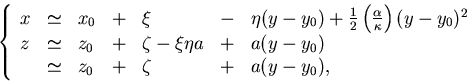(41)
where we have introduced the following short-hand for the helix parameters: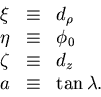Notice that the last approximation (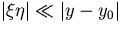), which is justified by our choice of the coordinate system (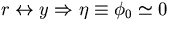), makes the r-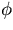and the r-z fittings decouple from each other. We will, thus, the r-and the r-z fittings separately in what follows. Notice also that the problem then becomes a linear one, which simplifies the necessary calculations considerably.

Keisuke Fujii
12/4/1998